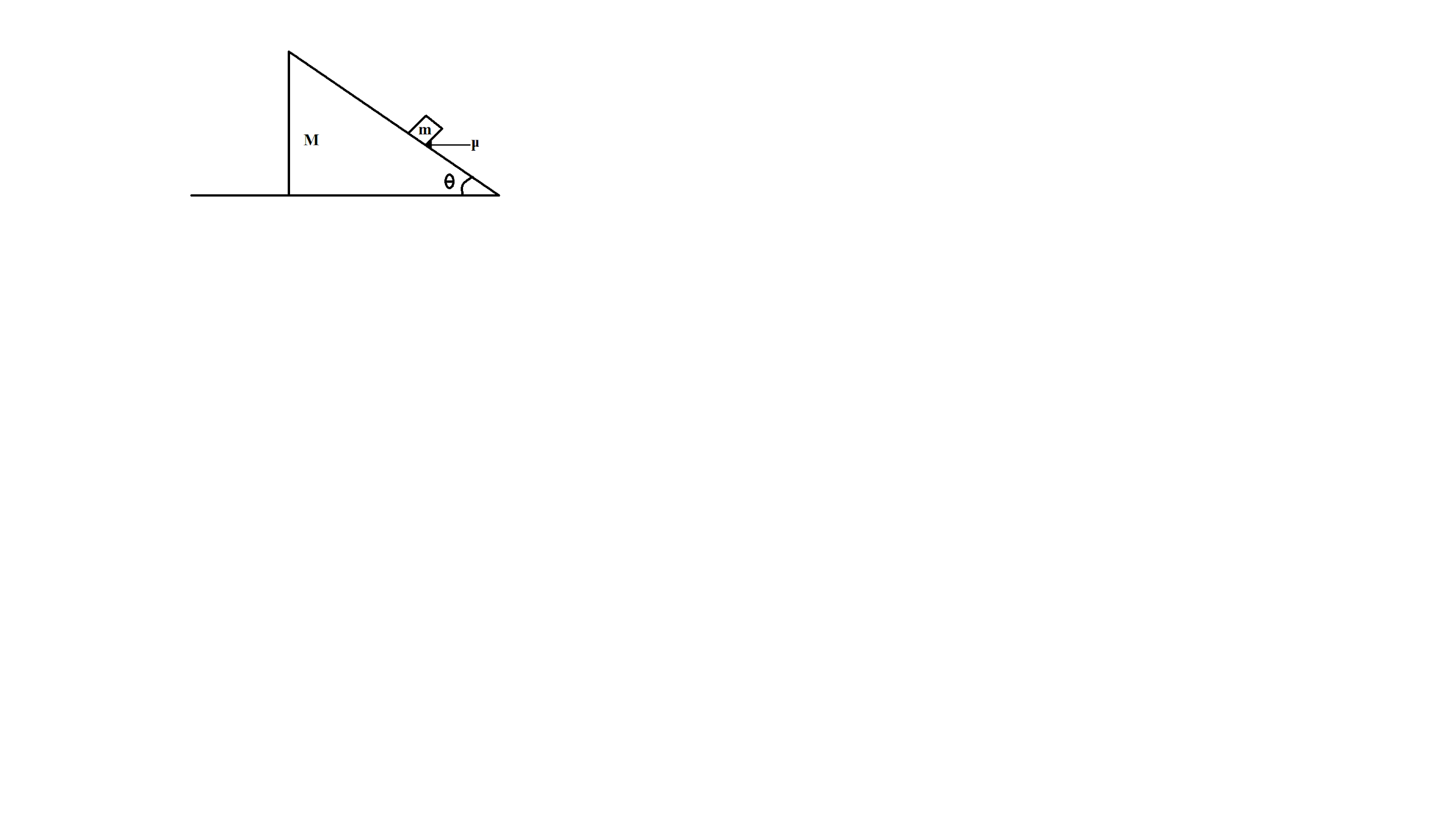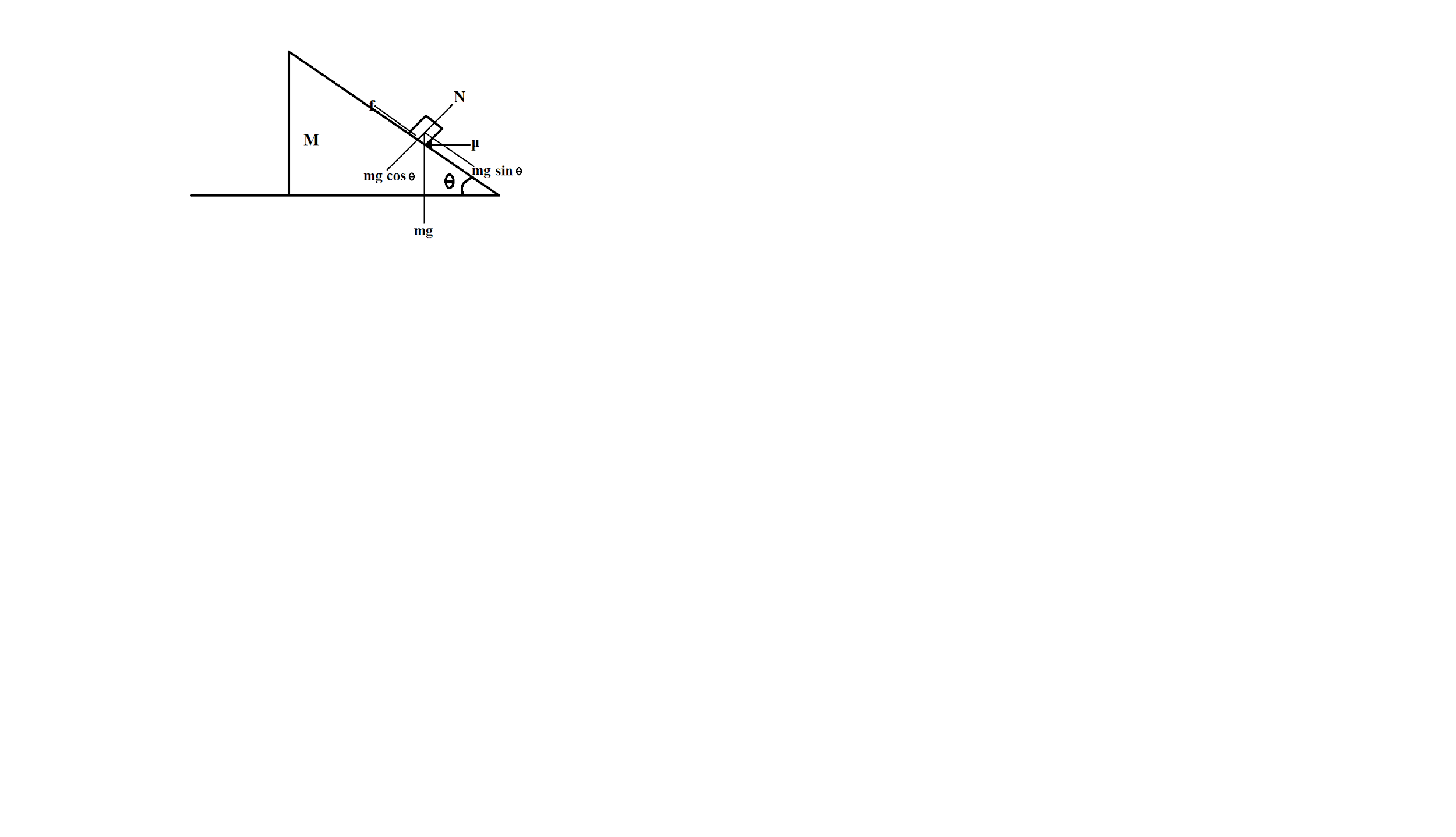QuestionAnswers

# Calculate angle of friction between wedge and block system is at rest. Coefficient of friction between the wedge and block is $\mu$ .\begin{align} & \text{A}\text{. ta}{{\text{n}}^{-1}}\mu \\ & \text{B}\text{. }2\theta \\ & \text{C}\text{. }\theta \\ & \text{D}\text{. }\dfrac{\theta }{2} \\ \end{align}Hint: For a block being placed on an inclined plane, different forces acting on the block are: Gravitational force, Normal force, and Frictional force. We will draw the force body diagram for the block and will determine the angle of friction between block and wedge. For the block to be at rest, all the forces acting on the block should be balanced, both in the direction parallel and perpendicular to the inclined plane.

Formula used:
Relation between frictional force and normal reaction,
$f=\mu N$

Motion of a block on an inclined plane is the interplay of different force types and the characterising features of the inclined plane. An inclined plane is a surface whose one end is raised. The raised surface forms angle $\theta$ with the horizontal. The block placed on the inclined surface is acted upon by the force of gravity and contact forces, normal force and frictional force.
Forces acting on the block of mass $m$ are:
Gravitational force $mg$ in downward direction,
Components of the gravitational force will be:
$mg\cos \theta$ in the direction parallel to the inclined plane and $mg\sin \theta$ in the direction perpendicular to the inclined plane
Frictional force $f$ in the direction opposite to the tending motion of the block
Force-body diagram (FBD) of block:As we are given that the system is at rest,
It means that all the forces on the block are balancing each other.
In direction perpendicular to the inclined plane, we have Normal force $N$ and component of gravitational force $mg\cos \theta$
These two forces will balance each other,
$N=mg\cos \theta$
In direction perpendicular to the inclined plane, we have frictional force $f$ and component of gravitational force $mg\sin \theta$
These two forces will balance each other,
$f=mg\sin \theta$
We have,
$f=\mu N$
Where,
$f$ is the frictional force
$\mu$ is the coefficient of friction
$N$ is the normal force
And, $N=mg\cos \theta$
\begin{align} & \mu N=mg\sin \theta \\ & \mu mg\cos \theta =mg\sin \theta \\ & \mu =\dfrac{\sin \theta }{\cos \theta } \\ & \mu =\tan \theta \\ & \theta ={{\tan }^{-1}}\mu \\ \end{align}
The angle of friction between the block and the wedge is ${{\tan }^{-1}}\mu$
Hence, the correct option is A.

Note: While making the force body diagram of an object placed on an inclined plane, all the forces should be considered and drawn carefully along the right direction. For an object to be at rest, the forces should be balanced in all the directions. Normal or reaction force always acts perpendicular to the surface of contact and frictional force is acted along the surface of contact.

View Notes
Relationship Between Force of Limiting Friction and Normal ReactionRolling Friction and Rolling ResistanceStatic FrictionRelation Between KVA and KWDifference Between RTD and ThermocoupleDifference Between Force and PowerDifference Between Diode and RectifierDifference Between Momentum and InertiaDifference Between Torque and PowerDifference Between Speed And VelocityCBSE Class 8 Science Friction WorksheetsImportant Questions for CBSE Class 11 Physics Chapter 11 - Thermal Properties of MatterImportant Questions for CBSE Class 8 Science Chapter 12 - FrictionImportant Questions for CBSE Class 11 Physics Chapter 7 - Systems of Particles and Rotational MotionImportant Questions for CBSE Class 12 Physics Chapter 11 - Dual Nature of Radiation and MatterImportant Questions for CBSE Class 11 PhysicsImportant Questions for CBSE Class 11 Physics Chapter 5 - Law of MotionImportant Questions for CBSE Class 11 Physics Chapter 9 - Mechanical Properties of SolidsImportant Questions for CBSE Class 11 Physics Chapter 2 - Units and MeasurementImportant Questions for CBSE Class 11 Physics Chapter 10 - Mechanical Properties of FluidsCBSE Class 12 Physics Question Paper 2020Previous Year Question Paper for CBSE Class 12 PhysicsPrevious Year Question Paper for CBSE Class 12 Physics - 2015Previous Year Question Paper for CBSE Class 12 Physics - 2018Previous Year Question Paper for CBSE Class 12 Physics - 2014Previous Year Question Paper for CBSE Class 12 Physics - 2013Previous Year Question Paper for CBSE Class 12 Physics - 2019Previous Year Physics Question Paper for CBSE Class 12 - 2017Physics Question Paper for CBSE Class 12 - 2016 Set 1 CPrevious Year Question Paper for CBSE Class 12 Physics - 2016 Set 1 SHC Verma Class 11 Physics Part-1 Solutions for Chapter 6 - FrictionFriction NCERT Solutions - Class 8 ScienceFriction NCERT Solutions - Class 8 ScienceNCERT Exemplar for Class 8 Science Solutions Chapter 12 FrictionClass 11 Physics NCERT solutionsNCERT Exemplar for Class 11 Physics Chapter 11 - Thermal Properties of Matter (Book Solutions)NCERT Solutions for Class 11 Physics Chapter 11 Thermal Properties of Matter in HindiNCERT Solutions for Class 8 Science Chapter 12 Friction in HindiNCERT Solutions for Class 12 Physics Chapter 11 Dual Nature of Radiation and Matter HindiNCERT Solutions for Class 11 Physics Chapter 11